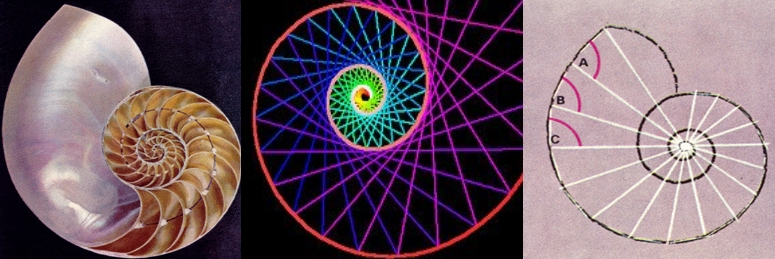# The mathematical beauty of the ShellsBehind the beautiful geometric spiral of the shell, there is a mathematical series known as the Fibonacci Succession, alias of the Italian mathematician Leonardo de Pisa (1170-1250). This sequence is possibly one of the best-known numerical sequences, given the properties it possesses and the large number of times it appears, in apparently unrelated matters. From the Fibonacci sequence derives, for example, the famous Golden Ratio or Golden Number, which is very frequently present in subjects related to architecture, art or nature itself.

The Fibonacci number series is obtained starting with the first two numbers, 0 and 1, and then each number in the series is obtained by adding the two that precede it, resulting in:

0, 1, 1, 2, 3, 5, 8, 13, 21, 34, 55, 89, 144, 233, 377, 610, 987, ...

These values ​​and the results of combining them appear very frequently in works of nature, but also, if in this series we divide each number that appears in it by the number that precedes it, a surprising result is obtained, and that is that as As we advance in the series, the result of the aforementioned divisions is tending, that is, it is getting closer and closer, to the value: 1.618034, which is precisely the Golden Ratio or Golden Number !, which is considered the standard of the perfect proportion in fields such as architecture, sculpture, painting and many others.

¡ Remember that when you buy a shell you buy an object with the perfect proportions created by nature !